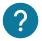# How to control an analog pin? How to control a component with AnalogWrite and AnalogRead?

This is what we will see in this course:

• Convert the value (map)

Here is the analog pins that you can control with analogWrite and analogRead:The analog is used to control the pin with PWM (pulse width modulation).

In contrast with the digital pins,  the analog pins don’t need pinMode to say if it’s an input or output.### What is the diference between Analog and Digital ?

A digital signal is a tension that can take only two values : HIGH or LOW. HIGH correspond to 5V and LOW to 0V. The digital signal is really useful to detect a state of a push button or to turn on a led.

Nevertheless if you want to turn on a Led at the half of tis power, you will need to use the analog signal.

This signal can take an infinite value between 0V an 5V. This signal will be useful for you if you want to have an accurate tension : 0.45V instead 0V or 5V for example.

## analogWrite ()

The analogwrite is used to control your component, such as a LED, servomotor. In fact, thanks to the PWM, the analogwrite can control your component with accuracy to give to position to a servomotor or a power to led.

``analogWrite(pin, value);``

The values with the PWM is for 0 to 255. 0 for 0V and 255 for 5V. You can choose a number between theses two values. Here is an example of the function AnalogWrite on the pin A3:

``analogWrite(A3, 122);``

If you want to read values from a sensor, you have to use the function analogRead(). Here is an example:

``analogRead(pin)``

pin : from A0 to A5 for an Arduino board.

The function return the value of the analog pin, which from 0 to 1023. We will see how to convert it.

## Value conversion

Map is a mathematical function which transform a value from an old range to a new one.

``map(value_to_convert, old_range_low, old_range_high, new_range_low, new_range_high);``
• analogWrite () : Convert value in % or in degree from 0 to 180°

In this part we will see different example of converting a value. In fact, the servomotor have to be controled from 0° to 180° and the LED have to be controled from 0% to 100%.

To control theses values to have a range from 0 to 255 we will use the function map.

For example, if we want the position 90° for our servomotor on the pin7, we write:

``````int value_servomotor=90;
value_servomotor=map(value_servomotor,0,180,0,255);
analogWrite(7,value_servomotor);``````
• analogRead () : Convert a value with a range from 0 to 1023 to a tension with a range between 0 to 5V

Here is an other example to convert the a value with a range from 0 to 1023 to a value with a range from 0V to 5V :

``````int valeur =analogRead(pin);
valeur=map(valeur,0,1023,0,5);
``````